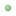[solved] Change poll sort order by highest, how?online0227Posts: 95 Threads: 56 Joined: Dec 2018 Reputation: 02021-06-07, 11:58 PM (This post was last modified: 2021-06-08, 12:28 PM by online0227. Edited 1 time in total.) Hi.. I am creating a small plugin that basically list polls in descending order in terms of the number of votes. In my plugin, I have: ``\$plugins->add_hook('showthread_end', 'poll_sort');`` and then the function, "poll_sort": ``````function poll_sort() { global \$mybb, \$db, \$lang, \$theme, \$templates, \$plugins, \$parser, \$cache, \$poll, \$option, \$polloptions, \$totpercent; \$lang->load("showthread"); \$thread = get_thread(\$poll['tid']); \$forum = get_forum(\$thread['fid']); \$forumpermissions = forum_permissions(\$forum['fid']); /* Basically copied and pasted from showthread.php but some parts are modified */ \$poll['timeout'] = \$poll['timeout']*60*60*24; \$expiretime = \$poll['dateline'] + \$poll['timeout']; \$now = TIME_NOW; // If the poll or the thread is closed or if the poll is expired, show the results. if(\$poll['closed'] == 1 || \$thread['closed'] == 1 || (\$expiretime < \$now && \$poll['timeout'] > 0) || \$forumpermissions['canvotepolls'] != 1) { \$showresults = 1; } if(\$forumpermissions['canvotepolls'] != 1) { \$nopermission = 1; } // Check if the user has voted before... if(\$mybb->user['uid']) { \$user_check = "uid='{\$mybb->user['uid']}'"; } else { \$user_check = "uid='0' AND ipaddress=".\$db->escape_binary(\$session->packedip); } \$query = \$db->simple_select("pollvotes", "*", "{\$user_check} AND pid='".\$poll['pid']."'"); while(\$votecheck = \$db->fetch_array(\$query)) { \$alreadyvoted = 1; \$votedfor[\$votecheck['voteoption']] = 1; } \$optionsarray = explode("||~|~||", \$poll['options']); \$votesarray = explode("||~|~||", \$poll['votes']); \$oldOrder = \$votesarray; arsort(\$votesarray); \$sortedOrder = \$votesarray; \$correctOrders = []; foreach(\$votesarray as \$key => \$value) { array_push(\$correctOrders, \$key); }; \$count = 0; foreach(\$votesarray as \$key => \$value) { \$newPoll[\$count] = \$optionsarray[\$key]; \$count++; }; \$optionsarray = \$newPoll; \$count = 0; foreach(\$votesarray as \$key => \$value) { \$newVotesArray[\$count] = \$value; \$count++; }; \$votesarray = \$newVotesArray; \$poll['question'] = htmlspecialchars_uni(\$poll['question']); \$polloptions = ''; \$totalvotes = 0; \$poll['totvotes'] = 0; for(\$i = 1; \$i <= \$poll['numoptions']; ++\$i) { \$poll['totvotes'] = \$poll['totvotes'] + \$votesarray[\$i-1]; } \$fillingOrder = 0; // Loop through the poll options. for(\$i = 1; \$i <= \$poll['numoptions']; ++\$i) { // Set up the parser options. \$parser_options = array( "allow_html" => \$forum['allowhtml'], "allow_mycode" => \$forum['allowmycode'], "allow_smilies" => \$forum['allowsmilies'], "allow_imgcode" => \$forum['allowimgcode'], "allow_videocode" => \$forum['allowvideocode'], "filter_badwords" => 1 ); if(\$mybb->user['showimages'] != 1 && \$mybb->user['uid'] != 0 || \$mybb->settings['guestimages'] != 1 && \$mybb->user['uid'] == 0) { \$parser_options['allow_imgcode'] = 0; } if(\$mybb->user['showvideos'] != 1 && \$mybb->user['uid'] != 0 || \$mybb->settings['guestvideos'] != 1 && \$mybb->user['uid'] == 0) { \$parser_options['allow_videocode'] = 0; } \$option = \$parser->parse_message(\$optionsarray[\$i-1], \$parser_options); preg_match('/\[uid=([0-9]*)]/', \$option, \$matches); if(strlen(\$matches > 0)) { \$country_cache = \$cache->read("countries"); \$poll_referred_uid = \$matches; \$poll_reffered_user = get_user(\$poll_referred_uid); \$poll_profile_link = get_profile_link(\$poll_reffered_user['uid']); \$poll_profile_image = (\$poll_reffered_user['avatar'] != null) ? \$poll_reffered_user['avatar'] : "./images/default_avatar.png"; \$poll_profile_country = \$country_cache[\$poll_reffered_user['country']]["flag"]; \$poll_profile_name = \$poll_reffered_user['username']; \$poll_profile = "  ".\$poll_profile_name." "; \$option = \$poll_profile; } if(\$i == 1) { \$option = "\u{1F947} ".\$option; } else if(\$i == 2) { \$option = "\u{1F948} ".\$option; } else if(\$i == 3) { \$option = "\u{1F949} ".\$option; } \$votes = \$votesarray[\$i-1]; \$totalvotes += \$votes; \$number = \$correctOrders[\$fillingOrder] + 1; \$fillingOrder++; // Mark the option the user voted for. if(!empty(\$votedfor[\$number])) { \$optionbg = "trow2"; \$votestar = "*"; } else { \$optionbg = "trow1"; \$votestar = ""; } // If the user already voted or if the results need to be shown, do so; else show voting screen. if(isset(\$alreadyvoted) || isset(\$showresults)) { if((int)\$votes == "0") { \$percent = "0"; } else { \$percent = number_format(\$votes / \$poll['totvotes'] * 100, 2); } \$imagewidth = round(\$percent); eval("\\$polloptions .= \"".\$templates->get("showthread_poll_resultbit")."\";"); } else { if(\$poll['multiple'] == 1) { eval("\\$polloptions .= \"".\$templates->get("showthread_poll_option_multiple")."\";"); } else { eval("\\$polloptions .= \"".\$templates->get("showthread_poll_option")."\";"); } } } // If there are any votes at all, all votes together will be 100%; if there are no votes, all votes together will be 0%. if(\$poll['totvotes']) { \$totpercent = "100%"; } else { \$totpercent = "0%"; } // Check if user is allowed to edit posts; if so, show "edit poll" link. \$edit_poll = ''; if(is_moderator(\$fid, 'canmanagepolls')) { eval("\\$edit_poll = \"".\$templates->get("showthread_poll_editpoll")."\";"); } // Decide what poll status to show depending on the status of the poll and whether or not the user voted already. if(isset(\$alreadyvoted) || isset(\$showresults) || isset(\$nopermission)) { if(\$alreadyvoted) { \$pollstatus = \$lang->already_voted; \$undovote = ''; if(\$mybb->usergroup['canundovotes'] == 1) { eval("\\$undovote = \"".\$templates->get("showthread_poll_undovote")."\";"); } } elseif(\$nopermission) { \$pollstatus = \$lang->no_voting_permission; } else { \$pollstatus = \$lang->poll_closed; } \$lang->total_votes = \$lang->sprintf(\$lang->total_votes, \$totalvotes); eval("\\$pollbox = \"".\$templates->get("showthread_poll_results")."\";"); \$plugins->run_hooks("showthread_poll_results"); } else { \$closeon = ' '; if(\$poll['timeout'] != 0) { \$closeon = \$lang->sprintf(\$lang->poll_closes, my_date(\$mybb->settings['dateformat'], \$expiretime)); } \$publicnote = ' '; if(\$poll['public'] == 1) { \$publicnote = \$lang->public_note; } eval("\\$pollbox = \"".\$templates->get("showthread_poll")."\";"); \$plugins->run_hooks("showthread_poll"); } }`````` Basically I copied and pasted from showthread.php to "poll_sort" function and altered it a little bit. It sorts polls using arsort, arsort(\$votesarray); Well this doesn't work as a plugin. But it worked when I altered the showthread.php directly. How do I achieve this as a plugin? or any tips Thank you very much. I tested hooks with many custom variable but it looks like only "showthread_start" allow me to use custom variable in template. Others hooks such as showthread_poll_results showthread_poll showthread_ismod showthread_threaded showthread_linear showthread_end They all do not allow me to use custom variable, and also any modification of \$options, \$polloptions, \$poll (all global variable) are all ignored, so that sorting doesn't work but show polls in original order. Solution All I had to do is to use "showthread_end" and return \$pollbox;.This \$pollbox should be global variable. If I use showthread_poll hook then it goes to infinite loop. So I used showthread_end hook instead. « Next Oldest | Next Newest »

Forum Jump:

Users browsing this thread: 1 Guest(s)